Question

Assume that a sample is used to estimate a population proportion p. Find the 90% confidence interval for a sample of size 248 with 120 successes. Enter your answer as a tri-linear inequality using decimals (not percents) accurate to three decimal places.

_________< p <__________

Solution :

Given that,

Point estimate = sample proportion =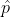= x / n = 120 / 248 = 0.484

1 -= 1 - 0.484 = 0..516

Z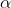/2 = 1.645

Margin of error = E = Z/ 2 *((* (1 -)) / n)

= 1.645 * (((0.484 * 0.516) / 248)

= 0.052

A 90% confidence interval for population proportion p is ,- E < p <+ E

0.484 - 0.052 < p < 0.484 + 0.052

0.432 < p < 0.536

#### Earn Coins

Coins can be redeemed for fabulous gifts.

Similar Homework Help Questions
• ### Assume that a sample is used to estimate a population proportion p. Find the 90% confidence...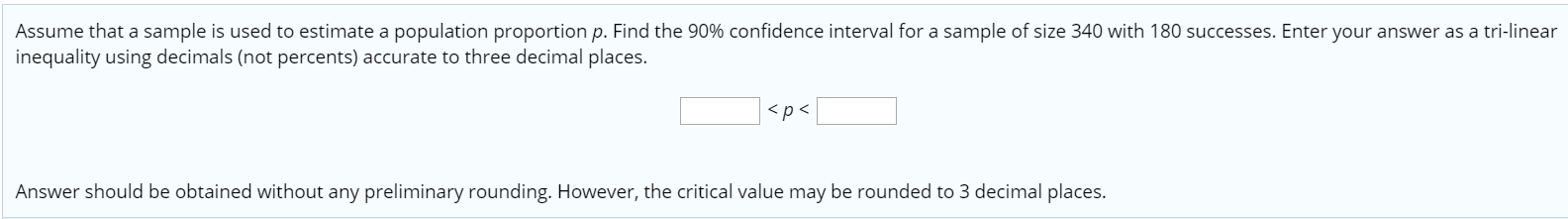Assume that a sample is used to estimate a population proportion p. Find the 90% confidence interval for a sample of size 340 with 180 successes. Enter your answer as a tri-linear inequality using decimals (not percents) accurate to three decimal places. <p> Answer should be obtained without any preliminary rounding. However, the critical value may be rounded to 3 decimal places.

• ### Assume that a sample is used to estimate a population proportion p. Find the 80% confidence...

Assume that a sample is used to estimate a population proportion p. Find the 80% confidence interval for a sample of size 227 with 112 successes. Enter your answer as a tri-linear inequality using decimals (not percents) accurate to three decimal places. ____< p < ____

• ### Assume that a sample is used to estimate a population proportion p. Find the 99.5% confidence...

Assume that a sample is used to estimate a population proportion p. Find the 99.5% confidence interval for a sample of size 82 with 73 successes. Enter your answer as a tri-linear inequality using decimals (not percents) accurate to three decimal places. < p < Answer should be obtained without any preliminary rounding. However, the critical value may be rounded to 3 decimal places.

• ### Assume that a sample is used to estimate a population proportion p. Find the 90% confidence...

Assume that a sample is used to estimate a population proportion p. Find the 90% confidence interval for a sample of size 279 with 38% successes. Enter your answer as an open - interval (i.e., parenthesis) using decimals (not percents) accurate to three decimal places. Confidence Interval = ______________.

• ### Assume that a sample is used to estimate a population proportion p. Find the 99.9% confidence...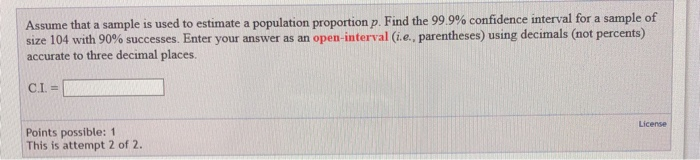Assume that a sample is used to estimate a population proportion p. Find the 99.9% confidence interval for a sample of size 104 with 90% successes. Enter your answer as an open-interval (i.e., parentheses) using decimals (not percents) accurate to three decimal places. C.I.= License Points possible: 1 This is attempt 2 of 2. Assume that a sample is used to estimate a population proportion p. Find the 99.9% confidence interval for a sample of size 104 with 90% successes....

• ### Assume that a sample is used to estimate a population proportion p. Find the 80% confidence...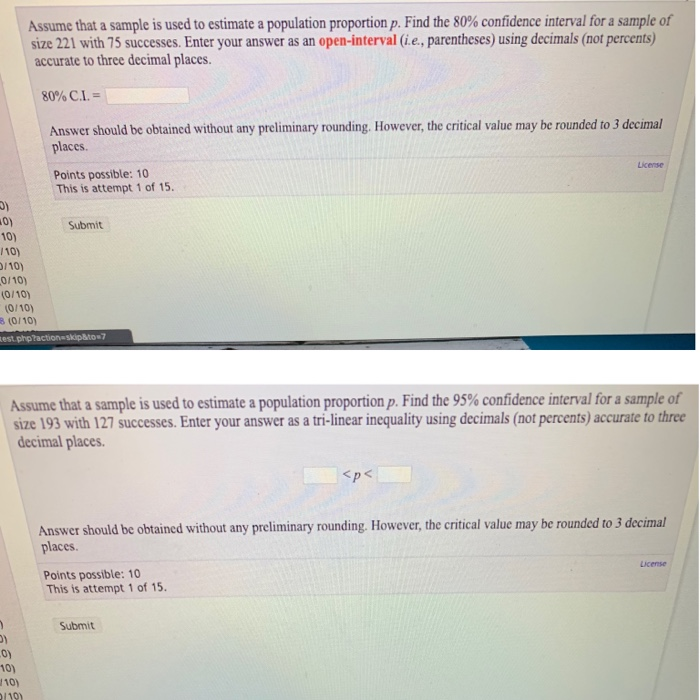Assume that a sample is used to estimate a population proportion p. Find the 80% confidence interval for a sample of size 221 with 75 successes. Enter your answer as an open-interval (i.e., parentheses) using decimals (not percents) accurate to three decimal places. 80% C.L. - Answer should be obtained without any preliminary rounding. However, the critical value may be rounded to 3 decimal places License Points possible: 10 This is attempt 1 of 15. Submit 10) 110) 1/10) 0/10)...

• ### Assume that a sample is used to estimate a population proportion p. Find the 80% confidence...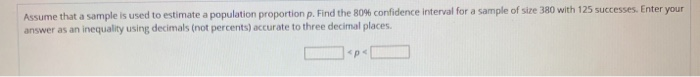Assume that a sample is used to estimate a population proportion p. Find the 80% confidence interval for a sample of size 380 with 125 successes. Enter your answer as an inequality using decimals (not percents) accurate to three decimal places. We wish to estimate what percent of adult residents in a certain county are parents. Out of 500 adult residents sampled, 195 had kids. Based on this, construct a 90% confidence interval for the proportion of adult residents who...

• ### Assume that a sample is used to estimate a population proportion p. Find the 90% confidence...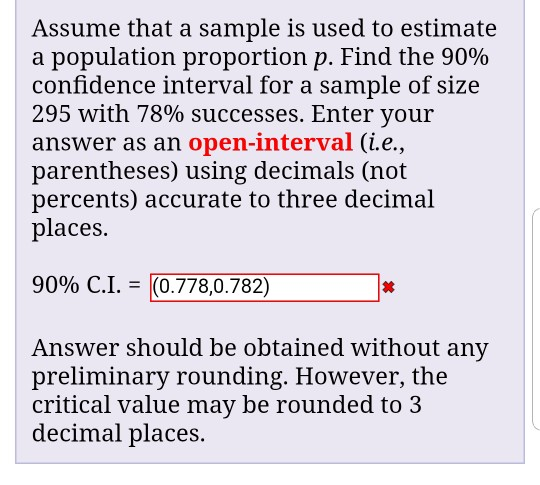Assume that a sample is used to estimate a population proportion p. Find the 90% confidence interval for a sample of size 295 with 78% successes. Enter your answer as an open-interval (i.e., parentheses) using decimals (not percents) accurate to three decimal places. 9090 C.1. = (0.778,0.782) 丼 Answer should be obtained without any preliminary rounding. However, the critical value may be rounded to 3 decimal places.

• ### Assume that a sample is used to estimate a population proportion p. Find the 99% confidence...

Assume that a sample is used to estimate a population proportion p. Find the 99% confidence interval for a sample of size 305 with 168 successes. Enter your answer as an open-interval (i.e., parentheses) using decimals (not percents) accurate to three decimal places. C.I. =

• ### Assume that a sample is used to estimate a population proportion p. Find the 95% confidence...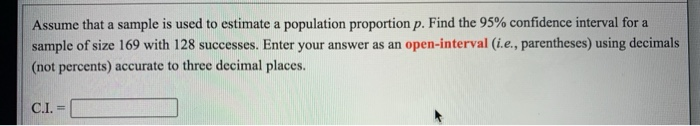Assume that a sample is used to estimate a population proportion p. Find the 95% confidence interval for a sample of size 169 with 128 successes. Enter your answer as an open-interval (i.e., parentheses) using decimals (not percents) accurate to three decimal places. C.I.